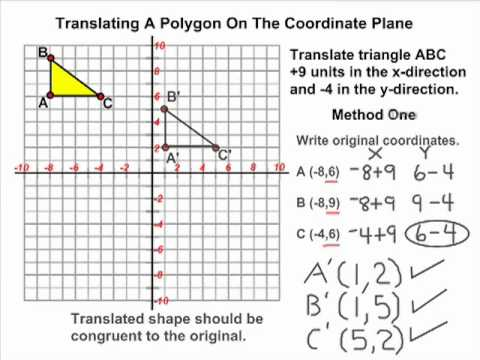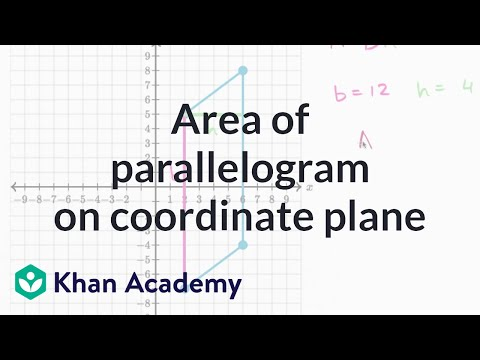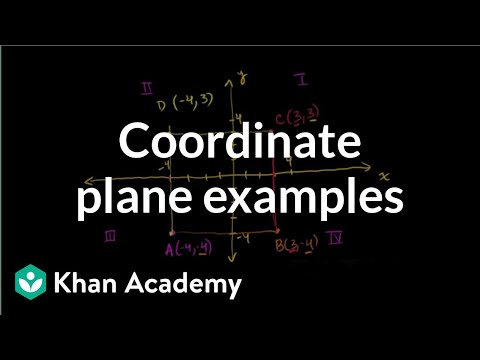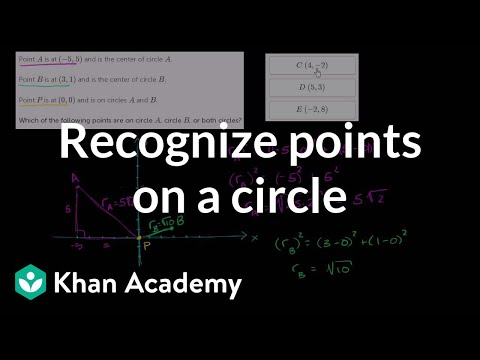# Dilation Of Objects On The Coordinate Plane

##By mrmaisonet

Dilation Of Objects On The Coordinate Plane# Transformations - Translating A Triangle On The Coordinate Plane

##By mrmaisonet

This tutorial reviews how to perform a translation on the coordinate plane using a triangle.# Geometry: 7-6 Dilations and Similarity in the Coordinate Plane

##By Sir Tyler Tarver

How to apply similarity properties in the coordinate plane and use a coordinate proof to prove figures similar. Yeah yeah.# Quadrilateral problems on the coordinate plane

##By Khan Academy

Learn how to find the area of a parallelogram on the coordinate plane.# The Coordinate Plane

##By MathVideos

This video covers the basics of coordinates planes such as: the /y/ and /x/ axes quadrants origin and ordered pairs. This is a good introduction.# Ex 1: Graph a Quadratic Inequality on the Coordinate Plane

##By Mathispower4u

Ex 1: Graph a Quadratic Inequality on the Coordinate Plane# Quadrilateral problems on the coordinate plane

##By Khan Academy

Sal solves a challenging problem involving a quadrilateral on the coordinate plane.# Parallelogram on the coordinate plane

##By Khan Academy

Remember our discussion of the coordinate plane? Sure you do! Let's graph the given coordinates of three of the polygon vertices and find where the 4th vertex is.# Points on the coordinate plane

##By Khan Academy

Let's get familiar with the x/y coordinate plane, both from the perspective of plotting points and interpreting the placement of points on a plane.# Points on the coordinate plane

##By Khan Academy

Let's get familiar with the x/y coordinate plane, both from the perspective of plotting points and interpreting the placement of points on a plane.# Points on the coordinate plane

##By Khan Academy

Let's get familiar with the x/y coordinate plane, both from the perspective of plotting points and interpreting the placement of points on a plane.# Points on the coordinate plane

##By Khan Academy

Let's get familiar with the x/y coordinate plane, both from the perspective of plotting points and interpreting the placement of points on a plane.# Points on the coordinate plane

##By Khan Academy

We're plotting an ordered pair on the x (horizontal) axis and y (vertical) axis of the coordinate plane.# Points on the coordinate plane

##By Khan Academy

We're plotting an ordered pair on the x (horizontal) axis and y (vertical) axis of the coordinate plane.# Points on the coordinate plane

##By Khan Academy

We're plotting an ordered pair on the x (horizontal) axis and y (vertical) axis of the coordinate plane.# Points on the coordinate plane

##By Khan Academy

We're plotting an ordered pair on the x (horizontal) axis and y (vertical) axis of the coordinate plane.# Quarter 2, Lesson 1: Coordinate Plane, Introduction to Graphing Linear Equations

##By Stan Lisle

In this video clip, the lesson reviews the coordinate planes and prepares the viewers to start graphing equations. The video provides clear examples with questions for viewers to answer after each lesson.# Coordinate plane word problems in the first quadrant

##By Khan Academy

Naomi needs some help counting the number of blocks she walks from home to school. We'll use the coordinate plane to graph it out first. Let's go.# Coordinate plane: graphing points word problem

##By Khan Academy

Naomi needs some help counting the number of blocks she walks from home to school. We'll use the coordinate plane to graph it out first. Let's go.# Geometry problems on the coordinate plane

##By Khan Academy

Watch Sal solve a challenging problem where he has to determine if points are on both, one, or neither of two circles.